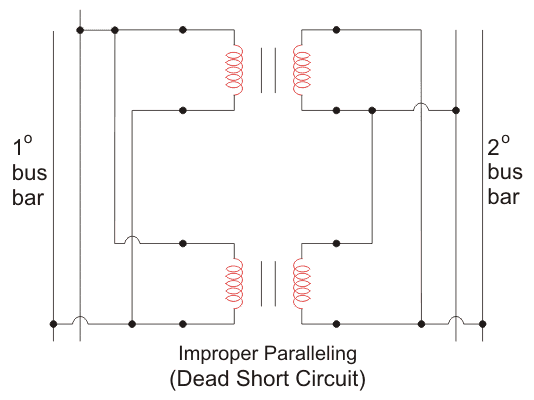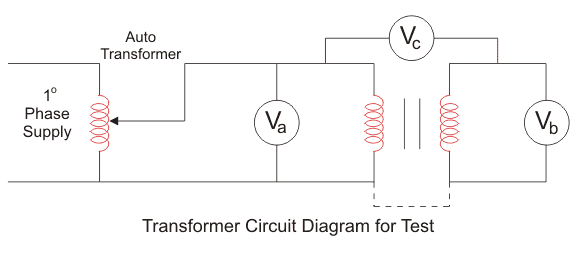Polarity Test of Transformer (Explanation + Diagrams)

Current flows from high voltage point to low voltage point due to the potential difference between them. Here, electrical polarity comes into the picture. Electrical polarity simply describes the direction of the current flow. When we look into DC system, we find that one pole is always positive and the other one is always negative that imply that the current flows in one direction only. But when we look into an AC system, the terminals are changing their polarity periodically, and the direction of the current also changes accordingly.

We use dot convention to identify the voltage polarity of the mutual inductance of two windings. The two used conventions are:

1. If a current enters the dotted terminal of one winding, then the voltage induced on the other winding will be positive at the dotted terminal of the second winding.
2. If a current leaves the dotted terminal of one winding, then the polarity of the voltage induced in the other winding will be negative at the dotted terminal of the second winding.

When we look into the operations of the distribution transformers, we find that they need to work all the time and also need to supply at high demand at peak times. So, to cope up with these situations, we connect the transformers in parallel.

Paralleling is done by connecting same polarity terminals of the primary winding together. A similar procedure is done for the secondary winding. Paralleling will increase the power supplying capacity and also the reliability of the system.We do polarity test on parallel transformers to ensure that we connect the same polarity windings and not the opposite ones. If we accidentally connect the opposite polarities of the windings, it will result in a short-circuit and eventually damage the machine.We can categorise the polarity of the transformer to two types,

2. Subtractive PolarityIn additive polarity, the voltage (Vc) between the primary side (Va) and the secondary side (Vb) will be the sum of both high voltage and the low voltage, i.e. we will get Vc = Va + Vb

Subtractive Polarity

In subtractive polarity, the voltage (Vc) between the primary side (Va) and the secondary side (Vb) will be the difference of both high voltage and the low voltage, i.e. we will get Vc = Va – Vb

In subtractive polarity, if Vc = Va – Vb, it is a step-down transformer and if Vc = Vb – Va, it is a step-up transformer.

We use additive polarity for small-scale distribution transformers and subtractive polarity for large-scale transformers.

Procedure of Polarity Test of Transformer1. Connect the circuit as shown above with a voltmeter (Va) across primary winding and another voltmeter (Vb) across the secondary winding.
2. If available, take down the ratings of the transformer and the turn ratio.
3. We connect a voltmeter (Vc) between primary and secondary windings.
4. We apply some voltage to the primary side.
5. By checking the value in the voltmeter (Vc), we can find whether it is additive or subtractive polarity.

If additive polarity – Vc should be showing the sum of Va and Vb.
If subtractive polarity – Vc should be showing the difference between Va and Vb.
Caution: Be careful that the max. measuring the voltage of voltmeter Vc should be greater than the sum of Va (Primary winding) and Vb (Secondary winding) otherwise during the additive polarity, the sum of Va and Vb comes across it.
Note: If we require additive polarity, but we have subtractive polarity, we can simply change it by keeping any of the primary or secondary windings in the same fashion and reversing the winding connection of the other one. Similarly, if we require subtractive polarity but have additive polarity, we could do the same procedure as above.

Want To Learn Faster? 🎓
Get electrical articles delivered to your inbox every week.
No credit card required—it’s 100% free.